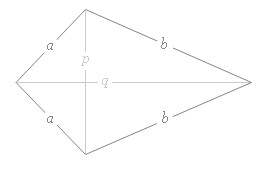## Kite Area Calculator

 Diagonal Length p= q = Result: Area of Kite: Square Unit

Kite Area Calculator to find out the Kite Area which uses the given diagonal length values of p and q.

### Kite area formulaKite Area = (1/2) x Diagonal p x Diagonal q

Kite Perimeter = 2a + 2b

Thinkcalculator.com provides you helpful and handy calculator resources.